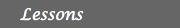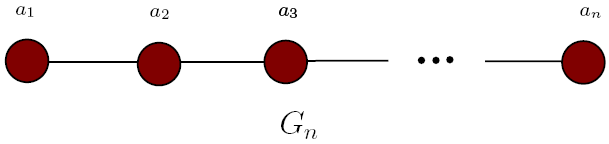MATH EXPLORERS' CLUB Cornell Department of Mathematics

# Ehrenfeucht-Fraïssé games

 Introduction1. History of EF games 2. Description 3. Examples 4. FO logic for graphs 5. EF games & FO logic 6. Applications References## Applications of EF games: Expressive power of FO logic

Ehrenfeucht-Fraïssé games have been traditionally used to prove inexpressiblity results for first order logic.
Metodology Theorem (Ehrenfeucht, Fraïssé): Given a property P, there is no first order sentence that expresses P, if and only if, for each positive integer n, one can find two graphs An and Bn such that:
1. property P is true on An
2. property P is false on Bn
3. A~nB, i.e. the Duplicator can win the n-move EF game on A and B.

## Example

A graph is said to be "even" if it has an even number of nodes. The property of a graph to be "even" is not expressible in first order logic.
proof: For each n, we consider the graphs An = G2n, and Bn = G2n+1, where G is depicted below:The Duplicator wins the n-moves EF game on An and Bn. Its winning strategy is simply that if the Spoiler chooses ai in the i-th round, the Duplicator chooses bi. (Compare this with the example in lesson 3! What is the difference?).
Exercise 1: A graph is called 2-colorable if one can color each node in either red or green such that no two adjacent nodes have the same color. Show that "2-colorability" is not expressible in first order logic.
hint: Think about the fact that the property that a graph has even number of nodes is not expressible in FO. How is this property related to 2-colorability?
Exercise 2: A graph is called Eulerian if it has an Eulerian cycle, i.e. a cycle that visits each node exactly once. Proove that the property of a graph of being Eulerian is not expressible in first-order logic.
hint: Look the following graph:Clearly, Cn is Eulerian if and only if n is an even number.
• So, for each n, choose An = C2n, and Bn = C2n+1.
• Show that the Duplicator wins the n-move EF game on An and Bn.
• Appy the Metodology Theorem to conclude that "Eulerian" is not expressible in first order logic.

 Top Next lesson